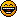Search on this siteSearch in forumsHome » Community » Newbie corner » [SOLVED] Cloning Array of complexe type
[SOLVED] Cloning Array of complexe type Wed, 25 March 2020 16:15Xemuth Messages: 179Registered: August 2018 Location: France Experienced Member
Hello community,

I'm wondering how can I copy an array of complexe type. See this example :
```#include <Core/Core.h>

using namespace Upp;

class A{
public:
int one = 1;
int two = 2;

virtual ~A(){}

virtual int sum()const =0;
virtual A* clone()const =0;
};

class B : public A{
public:
int three =3;

//Constructor
B(){}
B(const B& b){
one = b.one;
two = b.two;
three = b.three;
}
virtual ~B(){}

//Function
int sum()const{
return one +two +three;
}
B* clone()const {return new B(*this);}
};

class C : public A{
public:
int four =4;

//Constructor
C(){}
C(const C& c){
one = c.one;
two = c.two;
four = c.four;
}
virtual ~C(){}

//Function
int sum()const{
return one +two +four;
}
C* clone()const {return new C(*this);}
};

CONSOLE_APP_MAIN
{
ArrayMap<Upp::String, A> myArrays;
myArrays.Create<B>("FirstElement").one = 10;
myArrays.Create<B>("SecondElement").two =0;
myArrays.Create<C>("ThirdElement");

Cout() << myArrays.Get("FirstElement").sum() << EOL; //Correct print 15
Cout() << myArrays.Get("SecondElement").sum() << EOL; //Correct print 4
Cout() << myArrays.Get("ThirdElement").sum() << EOL; //Correct print 7

ArrayMap<Upp::String, A> aCopy(myArrays,myArrays.GetCount()); //not working
/*ArrayMap<Upp::String, A> aCopy;
aCopy = Upp::clone(myArrays); //Not working*/

myArrays.Clear(); //Clearing the base Array

Cout() << aCopy.Get("FirstElement").sum() << EOL; //If the copying goes well it should print 15 even if base array have been destroyed
Cout() << aCopy.Get("SecondElement").sum() << EOL;//If the copying goes well it should print 4 even if base array have been destroyed
Cout() << aCopy.Get("ThirdElement").sum() << EOL;//If the copying goes well it should print 7 even if base array have been destroyed
}
```

with this simple exemple, compilation error I get is "error: allocating an object of abstract class type 'A'" which makes sense.
Someone can explain me how can I do the trick and copy my array ?

[Updated on: Wed, 25 March 2020 21:13]

Report message to a moderator

Re: Cloning Array of complexe type [message #53275 is a reply to message #53269] Wed, 25 March 2020 18:34koldo Messages: 3129Registered: August 2008 Ultimate Member
This is not perfect, but I think it works.
(IMHO this is a little overcomplex for me)

```#include <Core/Core.h>

using namespace Upp;

#include <Core/Core.h>

using namespace Upp;

class A : MoveableAndDeepCopyOption<A> {
public:
int one = 1;
int two = 2;

A() {}
virtual ~A(){}

virtual int sum() const = 0;
A(const A& a, int) {
one = a.one;
two = a.two;
}

virtual A* clone()const = 0;
};

class B : public A {
public:
int three =3;

//Constructor
B(){}
B(const B& b, int){
one = b.one;
two = b.two;
three = b.three;
}
virtual ~B(){}

//Function
virtual int sum()const{
return one +two +three;
}
virtual B* clone()const {return new B(*this);}
};

class C : public A{
public:
int four =4;

//Constructor
C(){}
C(const C& c, int){
one = c.one;
two = c.two;
four = c.four;
}
virtual ~C(){}

//Function
virtual int sum()const{
return one +two +four;
}
virtual C* clone()const {return new C(*this);}
};

CONSOLE_APP_MAIN
{
ArrayMap<Upp::String, A> myArrays;
myArrays.Create<B>("FirstElement").one = 10;
myArrays.Create<B>("SecondElement").two =0;
myArrays.Create<C>("ThirdElement");

Cout() << myArrays.Get("FirstElement").sum() << EOL; //Correct print 15
Cout() << myArrays.Get("SecondElement").sum() << EOL; //Correct print 4
Cout() << myArrays.Get("ThirdElement").sum() << EOL; //Correct print 7

ArrayMap<Upp::String, A> aCopy;
for (int i = 0; i < myArrays.GetCount(); ++i)

myArrays.Clear(); //Clearing the base Array

Cout() << aCopy.Get("FirstElement").sum() << EOL; //If the copying goes well it should print 15 even if base array have been destroyed
Cout() << aCopy.Get("SecondElement").sum() << EOL;//If the copying goes well it should print 4 even if base array have been destroyed
Cout() << aCopy.Get("ThirdElement").sum() << EOL;//If the copying goes well it should print 7 even if base array have been destroyed
}```

Best regards
Iñaki
Re: Cloning Array of complexe type [message #53276 is a reply to message #53275] Wed, 25 March 2020 18:48koldo Messages: 3129Registered: August 2008 Ultimate Member
I think that in your case using directly clone() is problematic as it casts the child class constructor to the parent class.

Best regards
Iñaki

[Updated on: Wed, 25 March 2020 18:48]

Report message to a moderator

Re: Cloning Array of complexe type [message #53278 is a reply to message #53269] Wed, 25 March 2020 19:33Xemuth Messages: 179Registered: August 2018 Location: France Experienced Member
Hello Koldo, Thanks a lot for taking time to help me,

Your solution worked even without inherritance of A from MoveableAndDeepCopyOption.
But instead of doing this :
```ArrayMap<Upp::String, A> aCopy;
for (int i = 0; i < myArrays.GetCount(); ++i)
```

I'm surprised that ArrayMap don't have a simple function to do this.
However I have a little question, since My clone return a pointer to a static memory object wich is moved to the fresh object created by array,
what happen to this static allocation since I never delete anywhere this memory chunk ? Will it became a memory leak ?

Re: Cloning Array of complexe type [message #53279 is a reply to message #53278] Wed, 25 March 2020 19:58koldo Messages: 3129Registered: August 2008 Ultimate Member
Quote:
Will it became a memory leak ?

`virtual C* clone() const {return new C(*this);}`

Array:
```void Array<T>::Free() {
for(int i = 0; i < vector.GetCount(); i++)
delete (T *) vector[i];
}```

Best regards
Iñaki
Re: Cloning Array of complexe type [message #53280 is a reply to message #53279] Wed, 25 March 2020 20:08Xemuth Messages: 179Registered: August 2018 Location: France Experienced Member
Oh yes, I forget Add is not createPrevious Topic: ProgressIndicator issue Next Topic: Read txt, analyze and convert to csv
Goto Forum:

Current Time: Sat Jun 06 21:37:38 CEST 2020

Total time taken to generate the page: 0.00923 seconds